# Phase Shifter RMS Phase Error

We've seen the RMS phase calculation for a phase shifter screwed up so many times we want to present it here once and for all, in plain English! There are two traps that engineers fall into in this calculation, which we will identify. The main application for digital phase shifters and attenuators is in phased array systems.

New for December 2011: what is wrong with these pictures which were cropped from a datasheet from a certain MMIC supplier with a facility in Lowell, MA?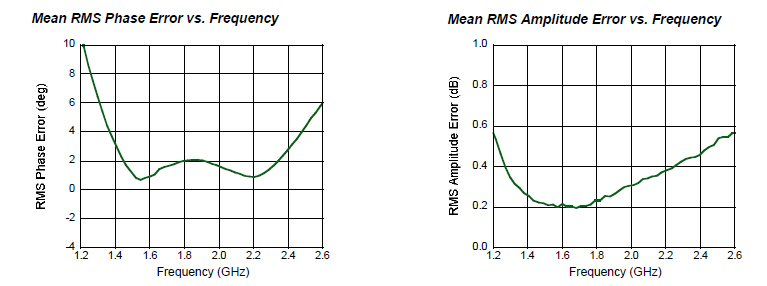What the heck is "Mean RMS" mean? How do you take the mean of a quantity that has already been squared, averaged and square-rooted? Perhaps data sheet editor should get one of these nice coffee mugs for Christmas...

But wait, here is a possible explanation from Martin:

I’m not employed by any for-profit organization, so this e-mail is not intended to give any more credit to the the datasheet makers then they deserve, but I would argue that a “Mean RMS Error” makes sense in the context of wafers containing zillions of MMICs which have, due to process variations, not necessarily the exact same performances. In that sense, when they measure, say, a hundred phase shifters, they can decide to put in their datasheet an average of the RMS Errors found over the population. Of course in my world, which is space, relying on this kind of figure is not a good idea, what I would like to see is the worst case across a certain temperature range, but then again, they might sell less, so they go with the more optimistic figure! Anyway, that’s my take on it…

That seems like a reasonable explanation, thanks! Now we will sit back and wait for the foundry to tell us that's what they meant... Now back to the discussion.

While we're on the subject, here's a trivia question... why are there 360 degrees in a circle? The same reason there are 60 minutes in an hour, and 24 hours in a day... because ancient mathematicians didn't have decimal digits, they wanted to use numbers that are divisible by as many smaller numbers as possible. They succeeded, 360 degrees is divisible by 2, 3, 4, 5, 6, 10, 12, 15, 18, 20, 24, etc!

An N-bit digital phase shifter has 2^N phase states. In almost most applications we are trying to control phase over 360 degrees. Therefore a two-bit phase shifter will have four phase states:

0, 90, 180, 270

A three bit phase shifter has eight phase states:

0, 45, 90, 135, 180, 225, 270, 315

A four bit phase shifter has 16 phase states:

0, 22.5, 45, 67.5, 90, 112.5, 135, 157.5, 180, 202.5, 225, 247.5, 270, 292.5, 315, 337.5

And so on. By the time you get six bits, the LSB is 5.625 degrees (360 divided by 64). Everyone knows you will never be able to split phase that accurately, so please refer to the lower order bits as 5.6, 11 and 22, not 5.625, 11.25 and 22.5, OK?

We've seen designs that go up to eight bits, but typically you will see digital phase shifters with three, four or five bits.

Calculating RMS phase error can be broken down into six easy steps:

1. Gather phase data and generate raw phase states
2. Compute phase errors of raw states
3. Compute average error (inverse of the "true" reference state)
4. Compute corrected phase states
5. Compute corrected phase errors
6. Perform root-mean-square of corrected phase errors

One more thing to keep in mind, which will add to your confusion at first. The convention that phase shifters follow is usually that the high-pass state is the reference state, and the low-pass state is the shifted state, which results in negative phase shift. But when the states are plotted or used in error calculations this convention is often thrown out the window because people don't like looking at a Y-axis that is negative. Now on with the calculation!

Let's give an example of a three-bit phase shifter. We faked some phase data for eight phase states and put it into Excel. It's not like TriQuint or M/A-COM supplies full data on their phase shifter products! The plot below shows eight transmission phase states (thanks to Yoko for correcting us when we couldn't count!) The downward slope of each phase state would be caused when phase shifter devices are measured in an electrically long fixture.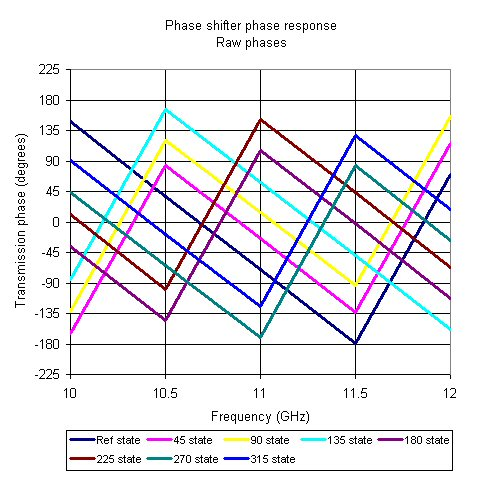Next we calculate seven raw phase states by subtracting the seven shifted states from the measured reference state (for now the "reference state" will be exactly zero degrees). But what happens if you merely subtract the phases of each state from the reference phase? You get a mess because the phases were all constrained to -180 and 180 degrees!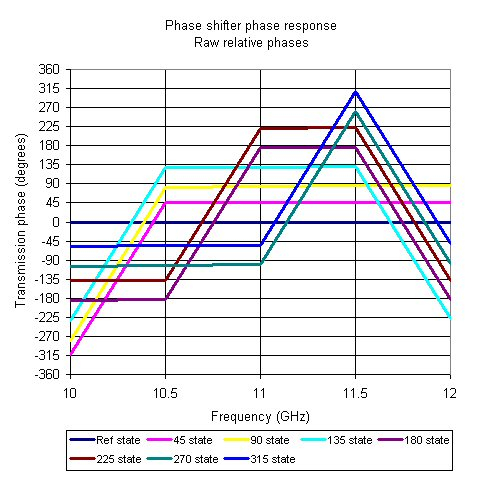There is more than one problem going on here. And more than one way to fix it. We recommend you use the "MOD" function on each of the phase states as well as the subtracted phase state:

Phase state 45 =MOD(MOD(45Phase,360)-MOD(RefPhase,360),360)

That's fixed it! Now we see the 45 bit is near perfect, while the 90 and 180 bits each fall short. The 315 state is the shortest, because it is constructed of the three bits, two of which were short. Dig?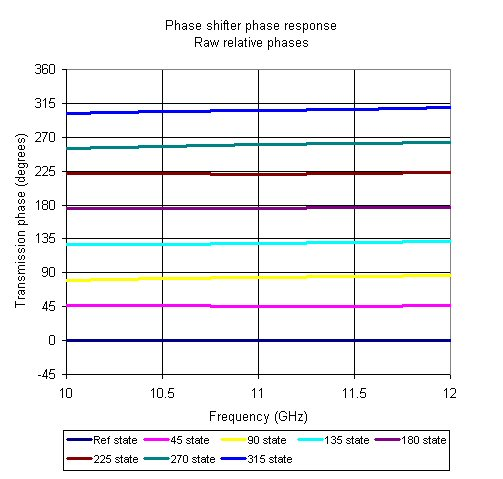Although it is possible to calculate an RMS error from the above plot, it would be wrong, not only that, it would be pessimistic. The problem is that the true reference state is not the measured reference state.

Now we'll calculate raw phase errors. This is done by subtracting the measured response above from the intended response. But let's first look at the intended response, the ideal three-bit phase shifter: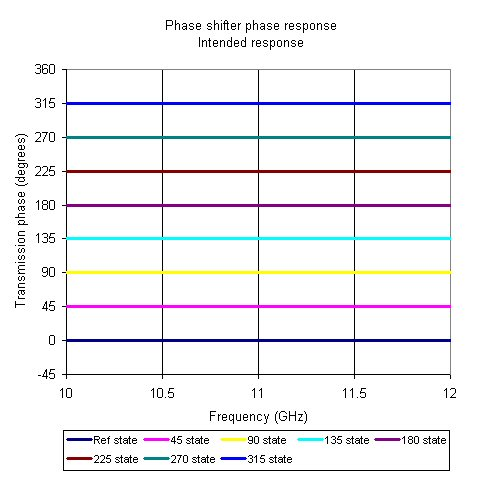The individual phase errors are found by subtracting the raw phase responses from the intended response. In the plot below we can see that the errors are predominantly negative, because two of the bits came up short. We've also plotted the average phase error (dashed line) which is the sum of the individual errors divided by the number of states, in this case divided by eight. Because the reference state has no phase error there is a tendency for engineers to divide the summed error by 2^N-1 (seven in this case) but that is WRONG!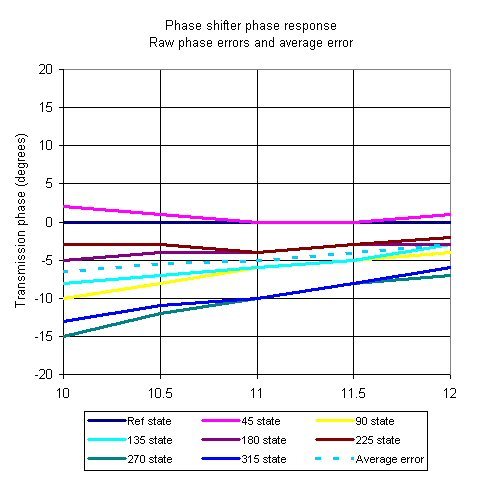Now we use the inverse of the average error (multiply it by -1) as our "true" reference state. Here's the corrected phase states, see how its coverage of 360 degrees is more even, but the reference state is no longer perfect! The 180 state is now nearly perfect instead of being short.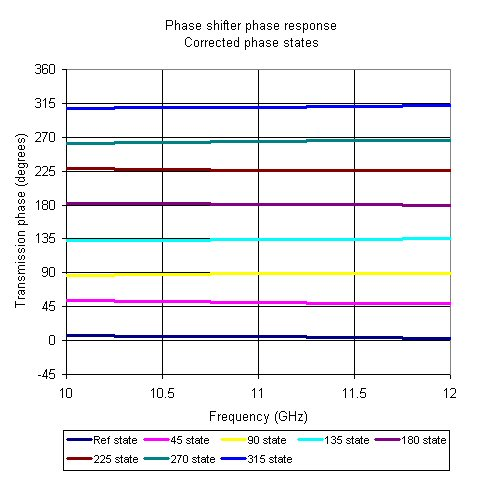Here's the corrected phase errors, now they are spread evenly about 0 degrees: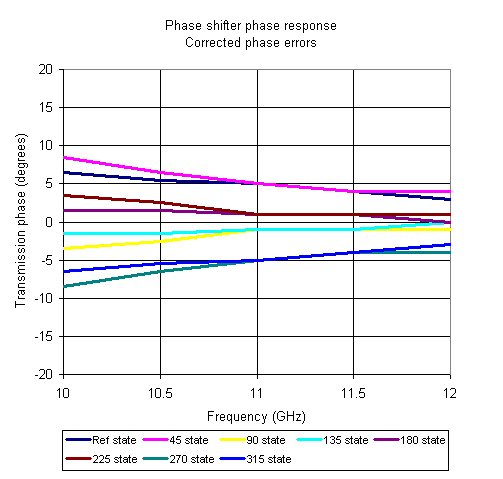And now we can finally calculate RMS error. This is done by squaring the errors of all eight phase states, summing them, dividing by the number of states (eight) and taking the square-root: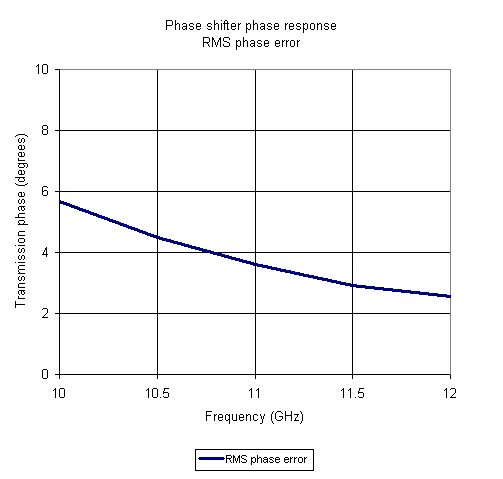The RMS error is highest at the lower frequency, which is obvious from the plots of individual phase errors. It is also (necessarily) less than the worst-case phase error at any frequency. It all makes sense!

If anyone wants a copy of the spreadsheet that we used to do this calculation, ask for it nicely and it's yours!